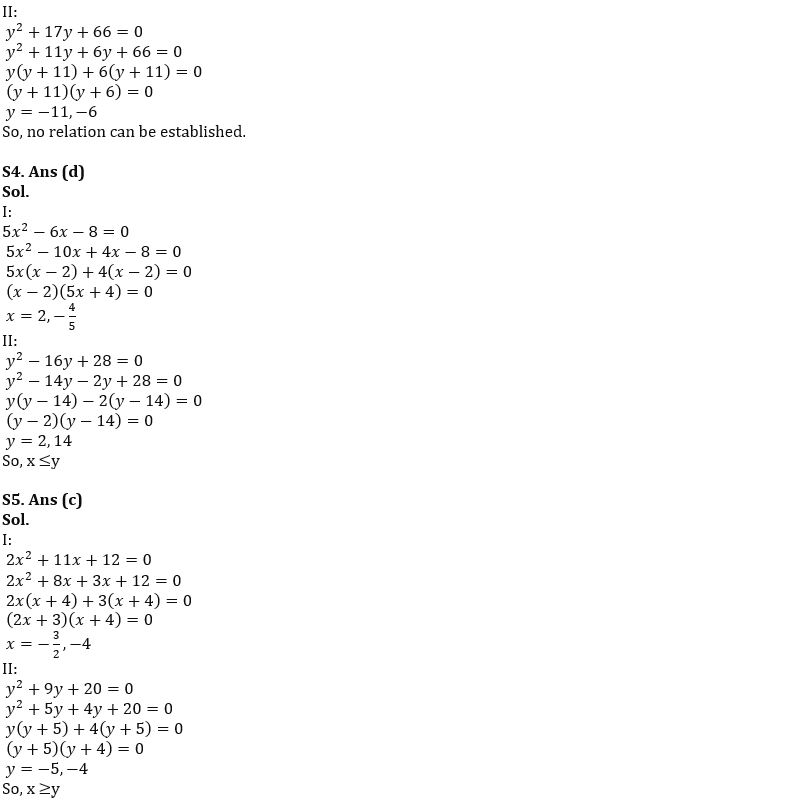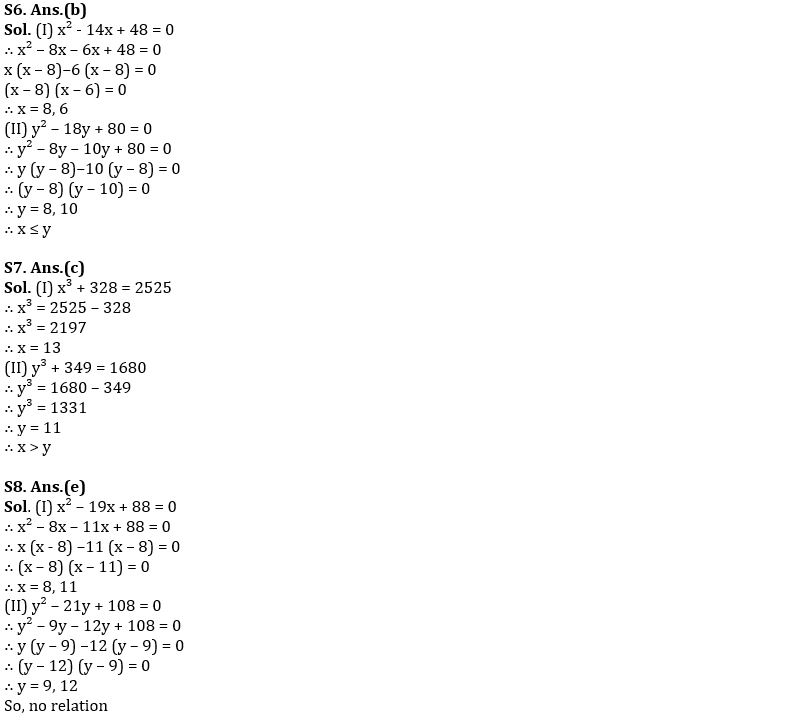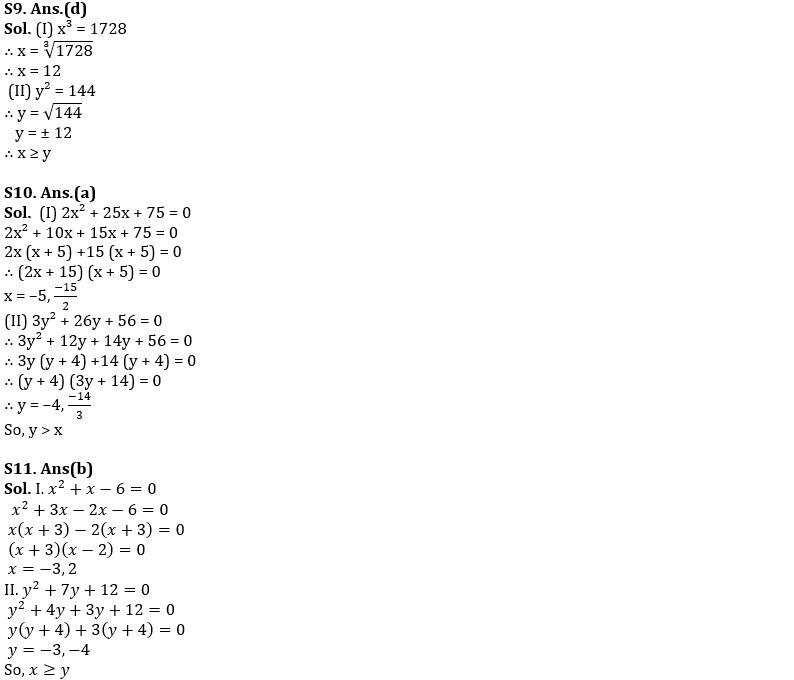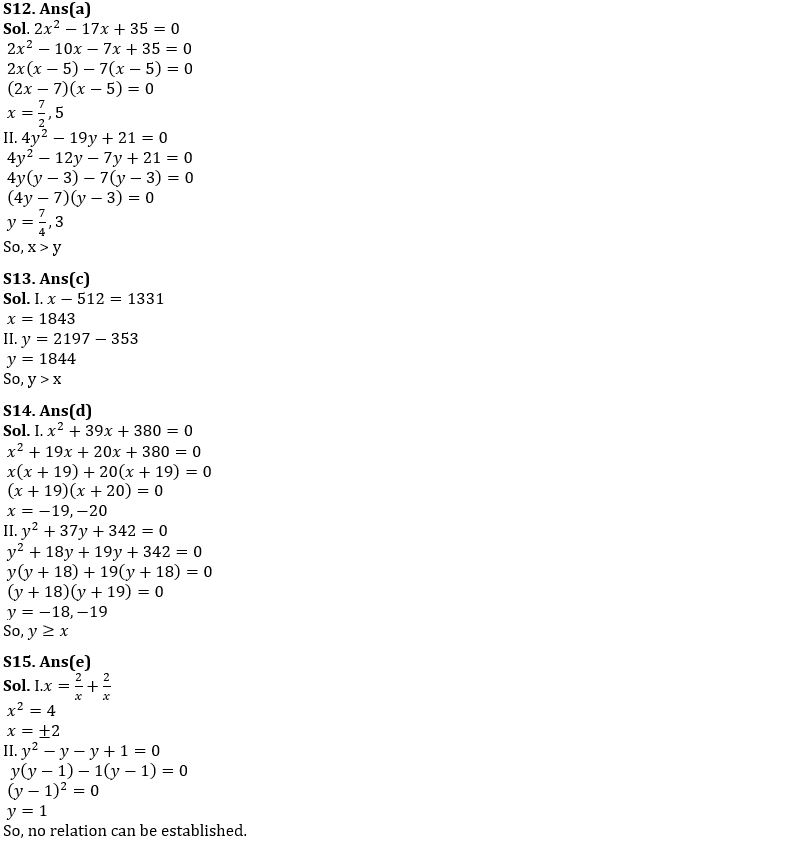Latest Banking jobs   »

# Quantitative Aptitude Quiz For FCI Phase I 2023- 3rd January

Directions (1-5): In each of the following questions, two equations (I) and (II) are given. Solve the equations and mark the correct option:
(a) if x>y
(b) if x<y
(c) if x≥y
(d) if x ≤y
(e) if x = y or no relation can be established between x and y.

Q1. I. 2x²-x-6=0
II. y²+9y+14=0

Q2. I. 3x²-8x+5=0
II. 2y²-15y+13=0

Q3. I. x²+3x-28=0
y²+17y+66=0

Q4. I. 5x²-6x-8=0
II. y²-16y+28=0

Q5. I. 2x²+11x+12=0
II. y²+9y+20=0

Directions (6-10): In each of the following questions two equations (I) and (II) are given, you have to solve both the equation and give answer.
(a) if x < y
(b) if x ≤ y
(c) if x > y
(d) if x ≥ y
(e) if x = y or no relationship can be obtained between x and y.

Q6. (I) x² – 14x + 48 = 0
(II) y² – 18y + 80 = 0

Q7. (I) x³ + 328 = 2525
(II) y³ + 349 = 1680

Q8. (I) x² – 19x + 88 = 0
(II) y² – 21y + 108 = 0

Q9. (I) x³ = 1728
(II) y² = 144

Q10. (I) 2x² + 25x + 75 = 0
(II) 3y² + 26y + 56 = 0

Directions (11-15): – In each of the following questions, two equations (I) and (II) are given, you have to solve both the equations and give answer.
(a) If x > y
(b) If x ≥ y
(c) If x < y
(d) If x ≤ y
(e) If x = y or no relation can be established between x and y.

Q11. I. x²+x-6=0
II. y²+7y+11=-1

Q12. I. 2x²-17x+35=0
II. 4y²-19y+21=0Solutions## FAQs

#### Congratulations!Union Budget 2023-24: Free PDF